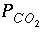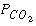| Next|| Previous || Top |

# Example 3.-- Mixing

This example demonstrates the capabilities of PHREEQC to perform a series of geochemical simulations, with the final simulations relying on results from previous simulations within the same run. The example investigates diagenetic reactions that may occur in zones where seawater mixes with carbonate ground water. The example is divided into five simulations, labeled A through E in table 15. (A) Carbonate ground water is defined by equilibrating pure water with calcite at aof 10 -2.0 atm. (B) Seawater is defined using the major-ion data given in table 10. (C) The two solutions are mixed together in the proportions 70 percent ground water and 30 percent seawater. (D) The mixture is equilibrated with calcite and dolomite. Finally, (E) the mixture is equilibrated with calcite only, to investigate the chemical evolution if dolomite precipitation is assumed to be negligible.

Table 15. --Input data set for example 3

```TITLE Example 3, part A.--Calcite equilibrium at log Pco2 = -2.0 and 25C.
SOLUTION 1  Pure water
pH      7.0
temp    25.0
EQUILIBRIUM_PHASES
CO2(g)          -2.0
Calcite         0.0
SAVE solution 1
END
TITLE Example 3, part B.--Definition of seawater.
SOLUTION 2  Seawater
units   ppm
pH      8.22
pe      8.451
density 1.023
temp    25.0
Ca              412.3
Mg              1291.8
Na              10768.0
K               399.1
Si              4.28
Cl              19353.0
Alkalinity      141.682 as HCO3
S(6)            2712.0
END
TITLE Example 3, part C.--Mix 70% ground water, 30% seawater.
MIX 1
1      0.7
2      0.3
SAVE solution   3
END
TITLE Example 3, part D.--Equilibrate mixture with calcite and dolomite.
EQUILIBRIUM_PHASES 1
Calcite         0.0
Dolomite        0.0
USE solution 3
END
TITLE Example 3, part E.--Equilibrate mixture with calcite only.
EQUILIBRIUM_PHASES 2
Calcite         0.0
USE solution 3
END
```

The input for part A (table 15) consists of the definition of pure water with SOLUTION input, and the definition of a pure-phase assemblage with EQUILIBRIUM_PHASES input. In the definition of the phases, only a saturation index was given for each phase. Because it was not entered, the amount of each phase defaults to 10.0 mol, which is essentially an unlimited supply for most phases. The batch reaction is implicitly defined to be the equilibration of the first solution defined in this simulation with the first pure-phase assemblage defined in the simulation. (Explicit definition of batch-reaction entities is done with the USE keyword.) The SAVE keyword instructs the program to save the batch-reaction solution composition from the final batch-reaction step as solution number 1. Thus, when the simulation begins, solution number 1 is pure water. After the batch-reaction calculations for the simulation are completed, the batch-reaction solution--water in equilibrium with calcite and CO 2 --is stored as solution 1.

Part B defines the composition of seawater, which is stored as solution number 2. Part C mixes ground water, (solution 1) with seawater (solution 2) in a closed system in whichis calculated, not specified. The MIX keyword is used to define the mixing fractions (approximately mixing volumes) of each solution in the mixture. The SAVE keyword causes the mixture to be saved as solution number 3. The MIX keyword allows the mixing of an unlimited number of solutions in whatever fractions are specified. The fractions (volumes) need not sum to 1.0. If the fractions were 7.0 and 3.0 instead of 0.7 and 0.3, the number of moles of each element in solution 1 (including hydrogen and oxygen) would be multiplied by 7.0, the number of moles of each element in solution 2 would be multiplied by 3.0, and the resulting moles of elements would be added together. The mass of water in the mixture would be approximately 10 kg (7.0 from solution 1 and 3.0 from solution 2) instead of approximately 1 kg, if the fractions were 0.7 and 0.3. The concentrations in the mixture would be the same for either set of mixing fractions because the relative proportions of solution 1 and solution 2 are the same. However, during subsequent reactions it would take 10 times more mole transfer for mixing fractions 7.0 and 3.0 than that shown in table 16 because there would be 10 times more water in the system.

Part D equilibrates the mixture with calcite and dolomite. The USE keyword specifies that solution number 3, which is the mixture from part C, is to be the solution with which the phases will equilibrate. By defining the phase assemblage with " EQUILIBRIUM_PHASES 1", the phase assemblage replaces the previous assemblage number 1 that was defined in part A. Part E performs a similar calculation to part D, but uses phase assemblage 2, which does not contain dolomite as a reactant.

Table 16. --Selected results for example 3

[Simulation A generates carbonate ground water; B defines seawater; C performs mixing with no other mole transfer; D equilibrates the mixture with calcite and dolomite; and E equilibrates the mixture with calcite only. Mole transfer is relative to the moles in the phase assemblage; positive numbers indicate an increase in the amount of the phase present, that is, precipitation; negative numbers indicate a decrease in the amount of the phase present, or dissolution. Saturation index: "--" indicates saturation index calculation not possible because one of the constituent elements was not in solution. Mole transfer: "--" indicates no mole transfer of this mineral was allowed in the simulation]

Simula-
tion

pH

logxx Saturation index x

xxx Mole transfer, millimoles xx

Calcite

Dolomite

CO 2

Calcite

Dolomite

A

7.297

-2.00

0.00

--

-1.976

-1.646

--

B

8.220

-3.38

.76

2.41

--

--

--

C

7.351

-2.23

-.10

.52

--

--

--

D

7.056

-1.98

.00

.00

--

-15.71

7.935

E

7.442

-2.31

.00

.73

--

-.040

--

Selected results from the output for example 3 are presented in table 16. The ground water produced by part A is in equilibrium with calcite and has a logof -2.0, as specified by the input. The moles of CO 2 in the phase assemblage decreased by about 2.0 mmol, which means that about 2.0 mmol dissolved into solution. Likewise, about 1.6 mmol of calcite dissolved. Part B defined seawater, which is calculated to have slightly greater than atmospheric carbon dioxide (-3.38 compared to about -3.5), and is supersaturated with calcite (saturation index 0.76) and dolomite (2.41). No mole transfers of minerals was allowed for part B. Part C performed the mixing and calculated the equilibrium distribution of species in the mixture, again with no mole transfers of the minerals allowed. The resulting logis -2.23, calcite is undersaturated, and dolomite is supersaturated. The saturation indices indicate that thermodynamically, dolomitization should occur, that is calcite should dissolve and dolomite should precipitate. Part D calculates the amounts of calcite and dolomite that should react. To produce equilibrium 15.71 mmol of calcite should dissolve and 7.935 mmol of dolomite should precipitate. Dolomitization is not observed to occur in present-day mixing zone environments, even though dolomite is the thermodynamically stable phase. The lack of significant dolomitization is due to the slow reaction kinetics of dolomite formation. Therefore, part E simulates what would happen if dolomite does not precipitate. If dolomite does not precipitate, only a very small amount of calcite dissolves (0.040 mmol) for this mixing ratio.

| Next|| Previous || Top |